Go back to Home

# Text analysis [R]

Data extraction and cleaning is very important and critical part of data analysis. Analysis is the easy part. Its the cleaning and extraction of raw data that takes time. One spends 80%-90% of the time in this process. Once that is done everyting else is quiet easy.

Recently I was given the task by a firm to extract text from a word document that consisted of abstract of different research articles in the field of healthare. Each abstract consisted of the following components:

1. Title of research article
2. Authors of research article
3. Institution of Authors
4. Abstract body

I had to extract these components from the word file and store the information in an excel format.

There were two excel files that I had to make

1) With three columns "s.no","abstract title","abstract"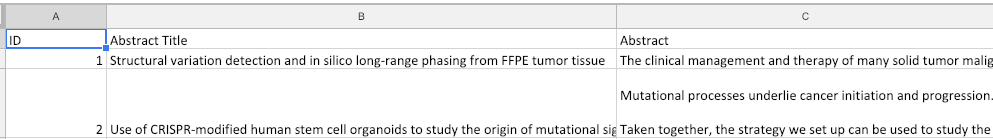2) With four columns "s.no","abstract title","Author","abstract".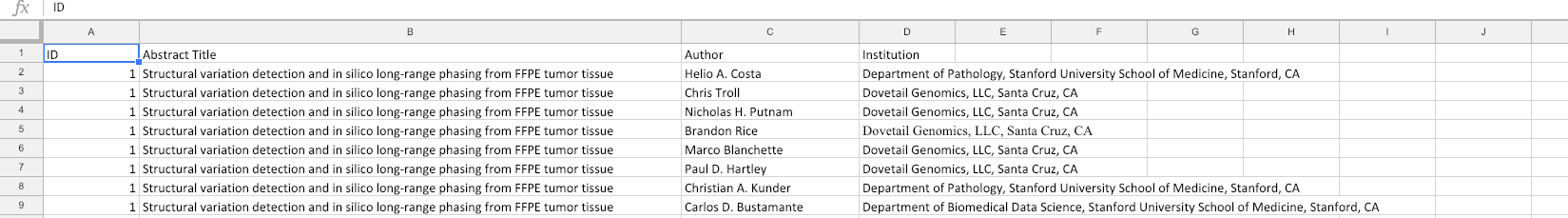The first task was to load the word file onto R. But after hours of searching online, I found one method to do so...but that didn't work. So I had to do someting else.

It is much easier to work on text file rather than word file in R. So I decided to save this file in .txt format. Little did I know, there are a lot of settings that needs to be changed when saving a word file to a text file. So after trying out different combination of settings, I choose these: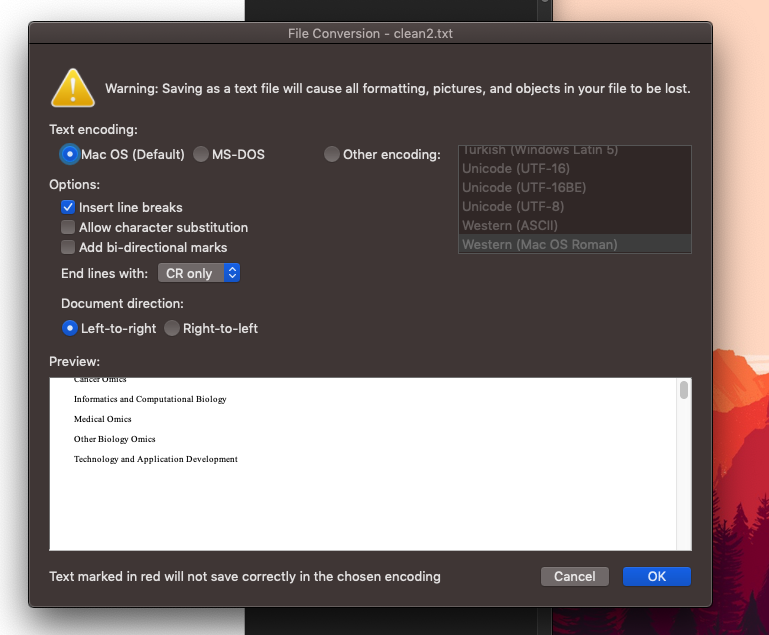Find the one that fits best for your needs.

After saving, "?" were added at the beginning as well as at the end of the abstract.(I think this denotes start of a new page in word).

This is how the text file looks now.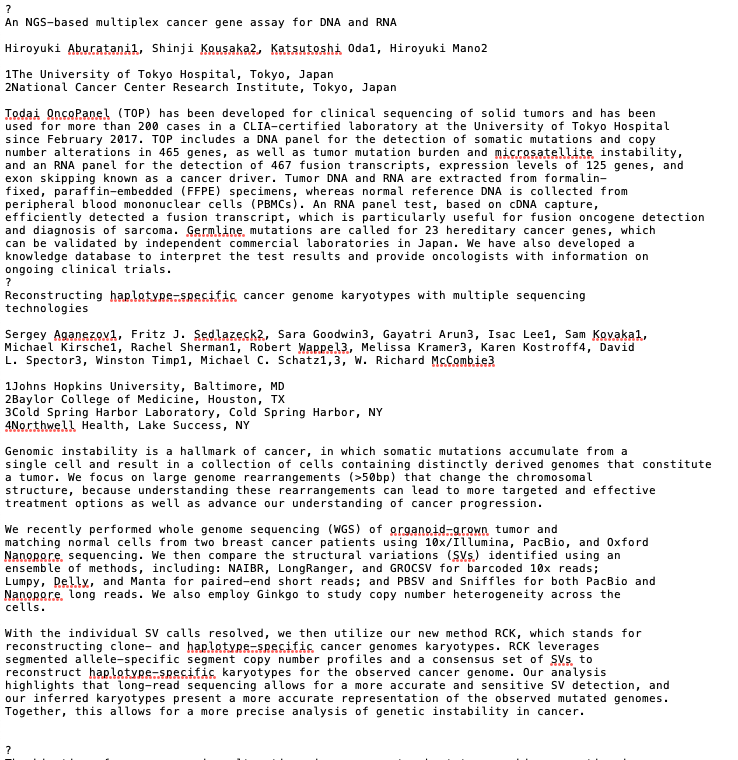## Data Extraction

Now starts the hard part, finding a pattern so that extraction of text can work for the whole document and not just the first abstract. This is necessary as it will help me apply regular expression to the code as well as help me decide the flow of my code.

NOW LET'S LOOK AT THE PATTERN I FOUNDGENERAL FLOW OF ONE ABSTRACT

1. Each abstract starts and ends with a "?"
2. After the first question mark "?" comes the TITLE of abstract
3. After TITLE comes an empty line(BLANK)
4. Followed by AUTHORS of the Abstract
5. After AUTHORS is another empty line(BLANK)
6. Then comes the INSTITUTIONS of the AUTHORS
7. Then again an empty line (BLANK)
8. Followed by the BODY of the abstract
9. Finally, a "?" marking the end of the abstract.
• TITLE: FIRST LINE
• AUTHOR: AFTER FIRST BLANK
• INSTITUTION: AFTER SECOND BLANK
• BODY: AFTER THIRD BLANK

You will notice a number after each author and a number before each institution. This denotes that the author belongs to the institute of that particular number.

Now that we have found the pattern, LETS START CODING!!

``````directory<-"/Users/priyanshumadan/Desktop"
file_name<-file.path(directory,"clean.txt")
• readLines() function reads each and every line of the text flie and save it as a large character "doc_lines

Next step is to find the positions of "?". I will use grep() function

``````marks<-grep("^\\?",doc_lines)
marks``````
• "?" has meaning in R. So to escape this I used "\\". "^" mean begining with. so the whole regular expression "^\\?" mean find the lines in doc_lines that start with a "?"

Now, I will create a list called "data". Each element of "data" will store all lines of an abstract.

``````#created list of abstracts "data"
list_element<-1
data<-list()
for (c in 1:length(marks)) {
fi<-c
en<-c+1
data[[list_element]]<-c(doc_lines[(marks[fi]+1):(marks[en]-1)])
list_element<-list_element+1
}``````
• The for loop will iterate along the length of the "marks" variable.
• "fi" is the first "?" and "en" is the next "?"
• "list_element" is a random variable that will iterate and store abstract in each element.
• data[[list_element]]<-c(doc_lines[(marks[fi]+1):(marks[en]-1)]) will store the lines between 2 consecutive "?"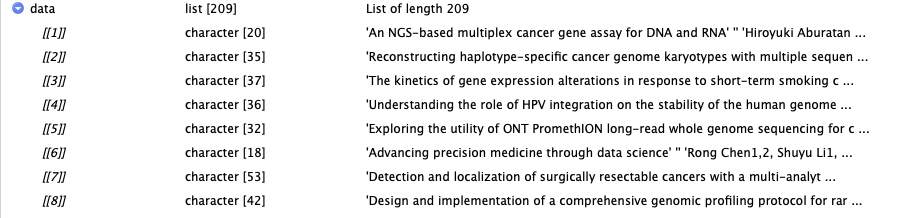This is how "data" list looks. As mention above each element stores each line of an abstract as a character.

Now I will create a data.frame "title_body". This will store the title of the abstract along with its abstract body.

``````title_body <- data.frame(matrix(nrow=length(data),ncol = 2))
colnames(title_body) <- c("title","body")

ro<-1
for (ro in 1:length(data)) {
start_blank<-(grep("^[:blank:]*\$|^[ ]*\$|^\t*\$",data[[ro]]))+1
end_blank<-length(data[[ro]])

title_start<-1
title_end<-grep("^[:blank:]*\$|^[ ]*\$|^\t*\$",data[[ro]])

title_con<-paste(c(data[[ro]][title_start:title_end]),collapse ="")
title_body\$title[ro]<-rbind(title_con)

body_con<-paste(c(data[[ro]][start_blank:end_blank]),collapse ="")
title_body\$body[ro]<-rbind(body_con)

}``````

For loop will be used to iterate along the length of the "data" list so as to extraxt title and body from each element of data.

As mention above, the pattern is:

• TITLE: FIRST LINE
• BODY: AFTER THIRD BLANK

First lets talk about the body of abstract.

So, start_blank looks for the third blank in the element after which the body starts and end_blank looks for end of the abstract. `Paste()` function combines all lines of the body and this is saved in body_con as a large character. body_con is then added to the body column of title_body.

Now the title of abstract.

title_start marks the start of the title which is the first line, and title_end marks the end of the title part which is the BLANK after title. `paste()` function is used to combine all lines of the title (if there is more than one line) and this is stored in title_con as a large character. title_con is then added to the title column of title_body.

NOTE: I forgot to add the s.no column and I realized that later when it was required.

``````title_body\$s.no<-NA
title_body\$s.no<-c(1:length(title_body\$s.no))``````

These lines add s.no column to the title_body

Moving on, Now I will create a data.frame called author_insti with 4 columns "s.no", "title", "author", "institution".

``````author_insti <- data.frame(matrix(nrow=length(data),ncol = 4))
colnames(author_insti) <- c("s.no","title","authors","institutions")

for (ro2 in 1:length(data)) {
start_blank_au<-(grep("^[:blank:]*\$|^[ ]*\$|^\t*\$",data[[ro2]]))+1
end_blank_au<-(grep("^[:blank:]*\$|^[ ]*\$|^\t*\$",data[[ro2]]))

author_con<-paste(c(data[[ro2]][start_blank_au:end_blank_au]),collapse ="")
author_insti\$authors[ro2]<-rbind(author_con)
author_insti\$s.no[ro2]<-ro2
}``````

For loop will be used to iterate along the length of the "data" list so as to extraxt author name from each element of data.

As mention above, the pattern is:

• AUTHOR: AFTER FIRST BLANK

So, start_blank_au looks for the first blank in the element after which the authors name starts and end_blank_au looks for end of the author names which is the second blank. `Paste()` function combines all lines of the authors and this is saved in author_con as a large character. author_con is then added to the author column of authoe_insti.

Now I will create one more data.frame institution_df that will store just the institution of each abstract

``````institutions_df <- data.frame(matrix(nrow=length(data),ncol = 1))
colnames(institutions_df) <- c("institutions")

ro3<-1
for (ro3 in 1:length(data)) {
start_blank_in<-(grep("^[:blank:]*\$|^[ ]*\$|^\t*\$",data[[ro3]]))+1
end_blank_in<-(grep("^[:blank:]*\$|^[ ]*\$|^\t*\$",data[[ro3]]))

in_con<-paste(c(data[[ro3]][start_blank_in:end_blank_in]),collapse =":")
institutions_df\$institutions[ro3]<-rbind(in_con)

}``````

As mention above, the pattern is:

• INSTITUTION: AFTER SECOND BLANK

So, start_blank_in looks for the second blank in the element after which the institutions name starts and end_blank_in looks for end of the institutions names which is the third blank. `Paste()` function combines all lines of the institutions and this is saved in in_con as a large character and each instittution name is seperated by ":" using "collapse" parameter in `paste()` function. This ":" help me differentiate one institution to another. in_con is then added to the institution column of institution_df.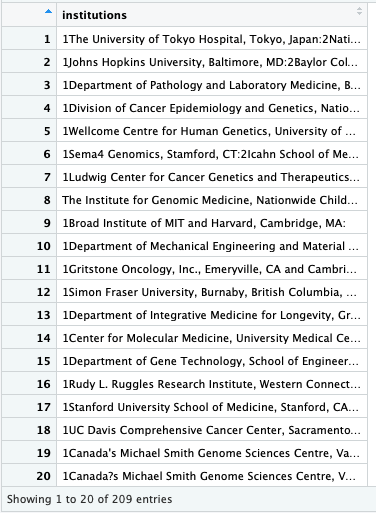Now I will create a list of all the authors of an abstract seperated by a "," and I will call this sep_author_list. I will besically split large character string in authors column in author_insti after every comma"," using `strsplit()` function

``````sep_author_list<-list()

for (aut_cnt in 1:length(data)) {
sep_author_list[[aut_cnt]]<-c(unlist(strsplit(noquote(author_insti\$authors[aut_cnt]),",")))
}``````

For loop will be used to iterate along the length of the "data" list.

Now I want to know the number of authors in each abstract. This can be done by counting the number of characters in each element of sep_author_list. I will store this count in a data.frame called insert_count with only one column called char_count

``````insert_count <- data.frame(matrix(nrow=length(data),ncol = 1))
colnames(insert_count) <- c("charac_count")

for (rows in 1:length(data)) {
insert_count\$charac_count[rows]<-length(sep_author_list[[rows]])
}``````

This is how inser_count dataframe looks. char_count column has number of author in each abstract.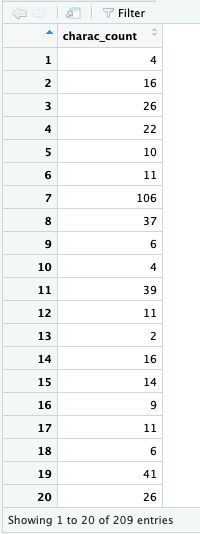I will use this data.frame to add rows to author_insti. The reason I am doing this so that I can add each individual author to a seperate row.

``````for (each in 1:length(insert_count\$charac_count)) {

for (in_row in 1:(insert_count\$charac_count[each]-1)) {
}

}``````

I have already given an explanation to these lines of code in my Inserting rows to data frame at any position learing post. Please have a look at that page for detailed explanation.

This is how author_insti dataframe looks now after inserting rows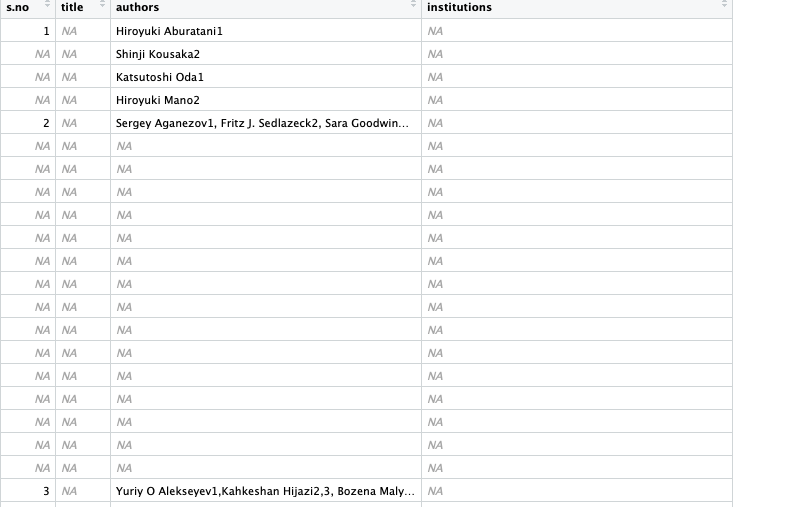Moving on...

Now that I have added blank rows after each, its time to add individual authors to each row.

``````for (sep in 1:length(insert_count\$charac_count)) {
author_insti\$authors[which(author_insti\$s.no==sep):(which(author_insti\$s.no==(sep+1))-1)]<-c(sep_author_list[[sep]])
}

#the authors of the last abstract are not added as the limit is reached, so it needs to be added manually.
author_insti\$authors[which(author_insti\$s.no==length(data)):length(author_insti\$authors)]<-c(sep_author_list[[length(data)]])``````

I have used s.no column in author_insti as my anchor points. What is happen in these lines of code is that I am asking R to insert values (in this case authors in each element of sep_author_list) between two consecutive s.no values.

For eg. if sep=1. Then I am using sep=1 to search the index of s.no=1 and index of row that is one before s.no=2. then I am using `c(sep_author_list[[sep]])` to add author names to rows between s.no=1 and s.no=2. this continues for all 209 abstracts.

Shortcoming of this is that the authors of last abstract don't get added in the column so i have to insert that manually using `author_insti\$authors[which(author_insti\$s.no==length(data)):length(author_insti\$authors)]<-c(sep_author_list[[length(data)]])`

This how the author_insti data.frame looks. you can see all the authors have their own rows. The cells that have just numbers are the authors that are in the previous cell. It denotes that the author belong to multiple institutions.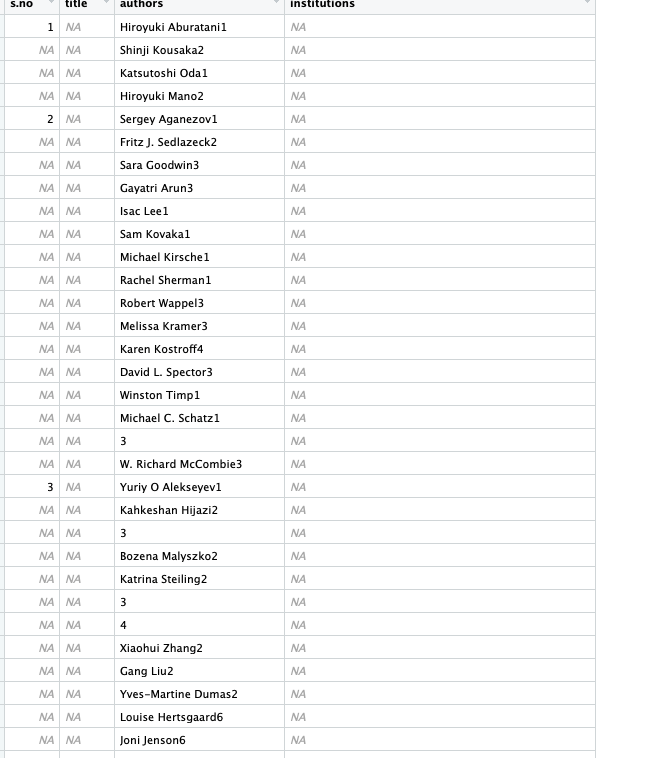Now that we are done with authors, we will now work on institutions. most abstracts may have more than one institutions and some might have only one. So we will seperate those. one data.frame will have only one institutions per abstract and the other that has multiple institution per abstract. The reason I am doing this is because single institutions can be added to author_insti directly next to their corresponding authors without any matching required.The rest will be similar to what we have done before with authors names. A little more work is required when we are dealing with more than one institution per abstract.

we will now create a data.frame multi_insti that will have name of institutions that are more than one per abstract.

``````temp_multi_insti <- data.frame(matrix(nrow=length(data),ncol = 2))
colnames(temp_multi_insti) <- c("s.no","institutions")
temp_multi_insti\$s.no[1:length(data)]<-c(1:length(data))
temp_multi_insti\$institutions<-institutions_df\$institutions

multi_insti<-temp_multi_insti[-c(which(ins_char_count\$ins_count==1)),]``````

I will do the same thing with single institutions

``````temp_single_insti<-data.frame(matrix(nrow=length(data),ncol = 2))
colnames(temp_single_insti) <- c("s.no","institutions")
temp_single_insti\$s.no[1:length(data)]<-c(1:length(data))
temp_single_insti\$institutions<-institutions_df\$institutions

single_insti<-temp_single_insti[c(which(all_ins_char_count\$ins_count==1)),]``````

Now I will insert institution names in single_insti to institution column in author_insti by matching s.no of both the data.frames.

``````for (sin_ins in 1:length(single_insti\$s.no)) {
author_insti\$institutions[which(author_insti\$s.no==single_insti\$s.no[sin_ins])]<-single_insti\$institutions[sin_ins]
}``````

Now that we are done with all the single institutions, lets work on multiple institutions.

As I did with the authors I will seperate all the institution in an element of multi_insti with a ":" and save it in a list called multi_institutions_list

``````multi_institutions_list<-list()

for (ins_cnt in 1:length(multi_insti\$institutions)) {
multi_institutions_list[[ins_cnt]]<-c(unlist(strsplit(noquote(multi_insti\$institutions[ins_cnt]),":")))
}``````

Now I will count the number of institutions in each element of list by using length function and save the count in ins_count column of ins_char_count data.frame.

``````ins_char_count <- data.frame(matrix(nrow=length(multi_institutions_list),ncol = 2))
colnames(ins_char_count) <- c("s.no","ins_count")
ins_char_count\$s.no<-multi_insti\$s.no

for (ins_rows in 1:length(multi_institutions_list)) {
ins_char_count\$ins_count[ins_rows]<-length(multi_institutions_list[[ins_rows]])
}``````

I will now create a data.frame called all_institutions with three columns "s.no",sub_num","institutions". I will explain the role of "sub_num" later on. I will insert all s.no values and then insert rows after every s.no based on the ins_char_count count

``````all_institutions <- data.frame(matrix(nrow=length(ins_char_count\$s.no),ncol = 3))
colnames(all_institutions) <- c("s.no","sub_num","institutions")
all_institutions\$s.no<-ins_char_count\$s.no

for (each_ins in 1:length(ins_char_count\$ins_count)) {

for (ins_in_row in 1:(ins_char_count\$ins_count[each_ins]-1)) {
}

}``````

Now that I have added rows to the data.frame for inserting institutions, I will now insert institutions the same way I added authors.

``````for (ins_sep in 1:length(ins_char_count\$ins_count)) {
all_institutions\$institutions[which(all_institutions\$s.no==ins_char_count\$s.no[ins_sep]):(which(all_institutions\$s.no==ins_char_count\$s.no[ins_sep+1])-1)]<-c(multi_institutions_list[[ins_sep]])
}

#the authors of the last abstract are not added as the limit is reached, so it needs to be added manually.
all_institutions\$institutions[which(all_institutions\$s.no==ins_char_count\$s.no[length(ins_char_count\$s.no)]):length(all_institutions\$institutions)]<-c(multi_institutions_list[[length(ins_char_count\$s.no)]])``````

Now as I added new rows, there are some blank spaces in s.no column of both author_insti and all_institutions. I will now fill those too.

``````for (s_num in 1:length(ins_char_count\$ins_count)) {
all_institutions\$s.no[which(all_institutions\$s.no==ins_char_count\$s.no[s_num]):(which(all_institutions\$s.no==ins_char_count\$s.no[s_num+1])-1)]<-ins_char_count\$s.no[s_num]
}

all_institutions\$s.no[which(all_institutions\$s.no==ins_char_count\$s.no[length(ins_char_count\$s.no)]):length(all_institutions\$s.no)]<-ins_char_count\$s.no[length(ins_char_count\$s.no)]

for (s_num2 in 1:length(data)) {
author_insti\$s.no[which(author_insti\$s.no==s_num2):(which(author_insti\$s.no==(s_num2+1))-1)]<-s_num2
}

author_insti\$s.no[which(author_insti\$s.no==length(data)):length(author_insti\$s.no)]<-length(data)``````

This is how s.no column should look like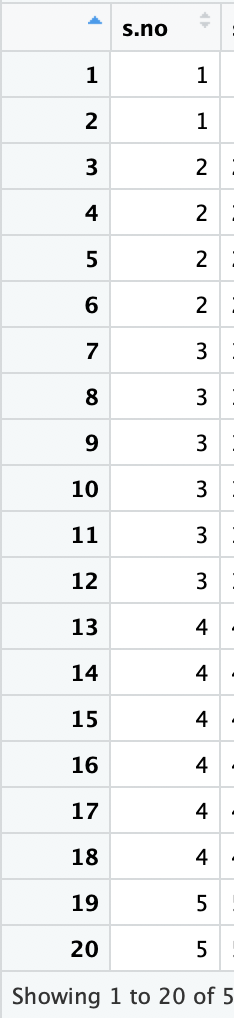NOW I REALLY ENJOYED DOING WHAT I DID NEXT.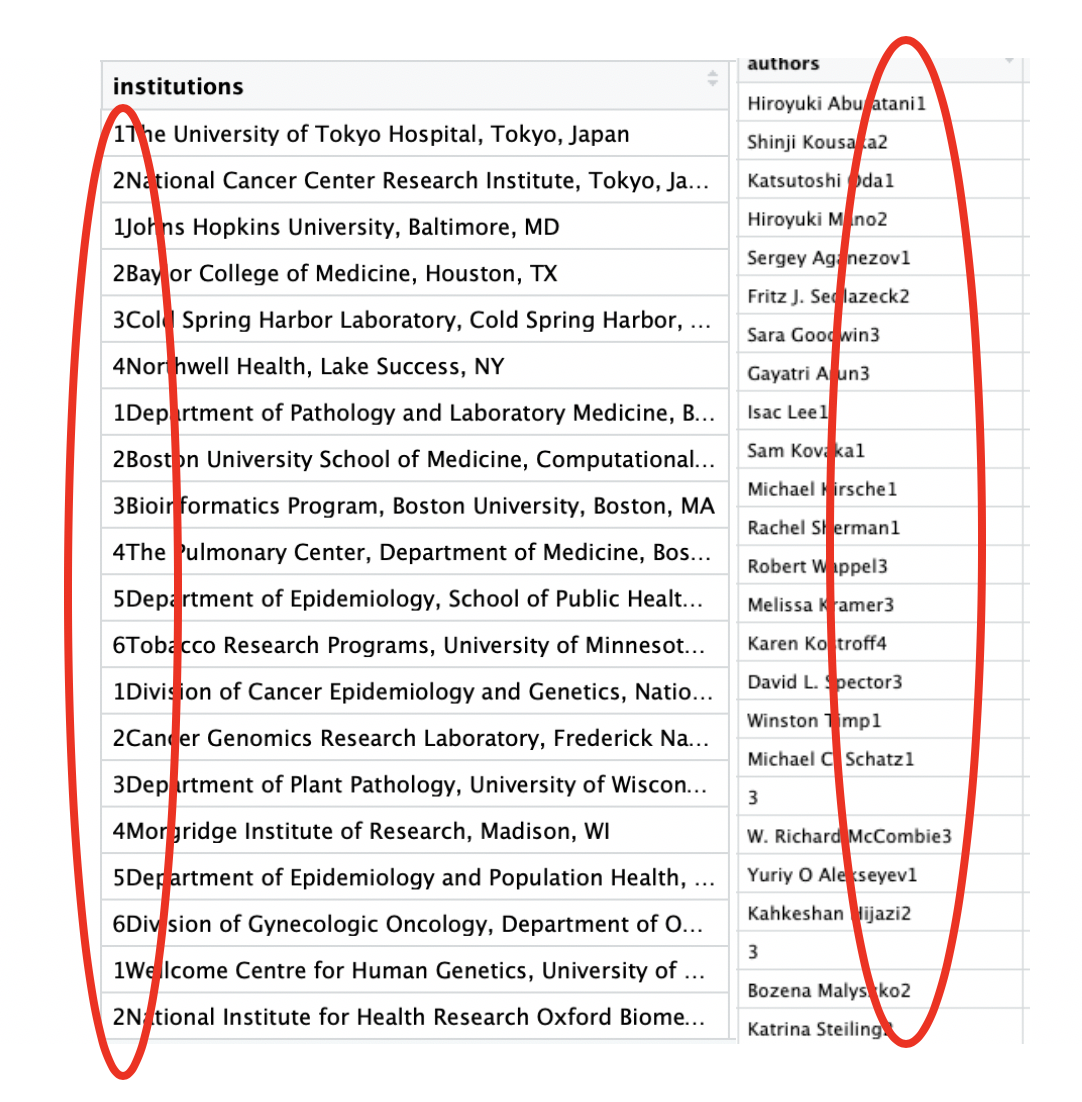As you can see the numbers next to author names and numbers befor institution names, I am going to extract these numbers using regexec() function. I will save numbers in authors column to auth_num column in author_inst and numbers in institution to ins_num column of all_institution.

``````##################################### Extract number from author names###############################
author_num<-regexec("[[:digit:]]+\$",author_insti\$authors)
author_num
auth_num_match_list<-regmatches(author_insti\$authors,author_num)
auth_num_match_list
author_insti\$auth_num<-NA
author_insti\$auth_num<-auth_num_match_list
#################################### Extract numbers from institutions names #############################
ins_num<-regexec("^[[:digit:]]+",all_institutions\$institutions)
ins_num
ins_num_match_list<-regmatches(all_institutions\$institutions,ins_num)
ins_num_match_list
all_institutions\$in_num<-NA
all_institutions\$in_num<-ins_num_match_list``````

Now I have to create a unique ID for both authors as well as Institutions so that I can combine the authors to their corresponding institutions. "sub_num" in both these data frames author_insti and all_institutions will work as an unique ID.

This is how it is going to work. I will combine s.no to auth_num in author_insti and to ins_num in all_institution using .

``````#################################paste s.no to in_num ################################################

for (i in 1:length(all_institutions\$s.no)) {
all_institutions\$sub_num[i]<-paste(as.character(all_institutions\$s.no[i]),all_institutions\$in_num[[i]],sep = ".")
}

#################################paste s.no to auth_num ################################################

author_insti\$sub_num<-NA

for (j in 1:length(author_insti\$s.no)) {
author_insti\$sub_num[j]<-paste(as.character(author_insti\$s.no[j]),author_insti\$auth_num[[j]],sep = ".")
}``````

for eg. if s.no=1 and auth_num=2 then sub_num=1.2

This is how the sub_num column looks in all_institution. Similar is the case in author_insti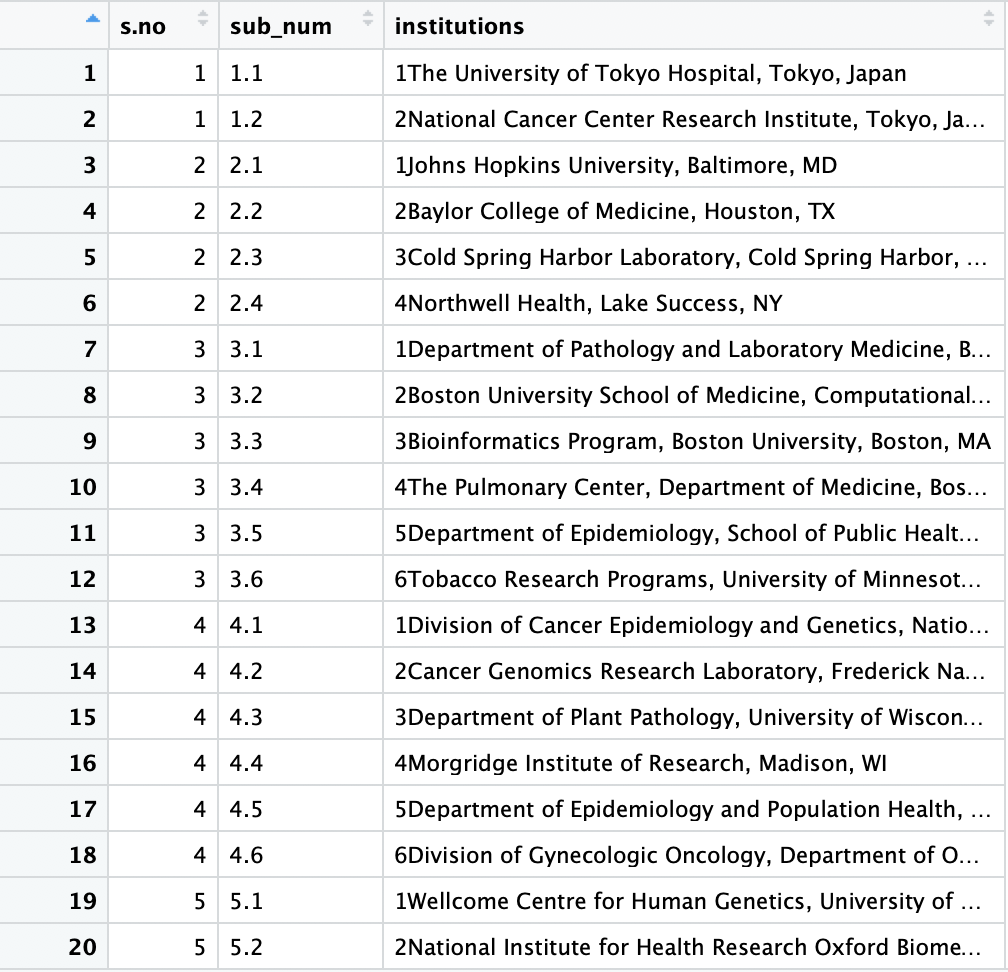Now I will match the "sub_num" of both the dataframes and add institution from all_institution to institution column of author_insti when "sub_num" are same in both table.

``author_insti\$institutions<-all_institutions\$institutions[match(author_insti\$sub_num,all_institutions\$sub_num)]``

After this I replaced the cells with just numbers with author names. And made necessary changes that had to be done manually by writing a seperate code or by making edits to the text file itself.

``````rep_name<-grep("^[[:digit:]]+",author_insti\$authors)
author_insti\$authors[rep_name[1:length(rep_name)]]<-author_insti\$authors[(rep_name[1:length(rep_name)]-1)]``````

Now finally remove all the number in the author and institution column of author_insti

``````grep("[[:digit:]]+\$",author_insti\$authors)

author_insti\$authors<-gsub("[[:digit:]]+\$","",author_insti\$authors)
author_insti\$institutions<-gsub("^[[:digit:]]+","",author_insti\$institutions)``````

AND WE ARE DONE!!!!!

This is the final file. Clean anmd ready for analysis. :)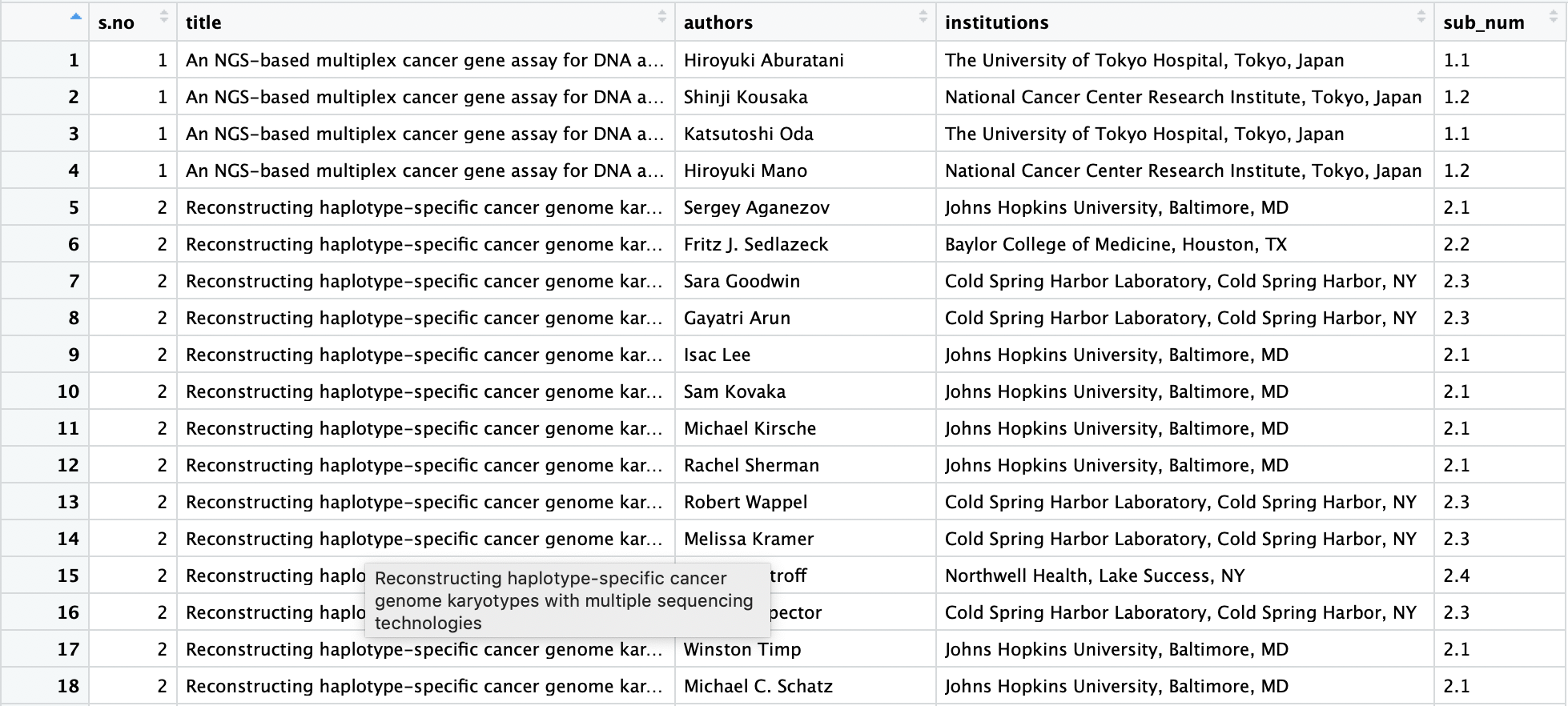LAST THING TO DO IS SAVE THIS AS A CSV FILE

``````write.csv(title_body,'title_and_body_final.csv')
write.csv(author_insti,'author_insti_final.csv')
write.csv(all_institutions,'institute_list.csv')``````